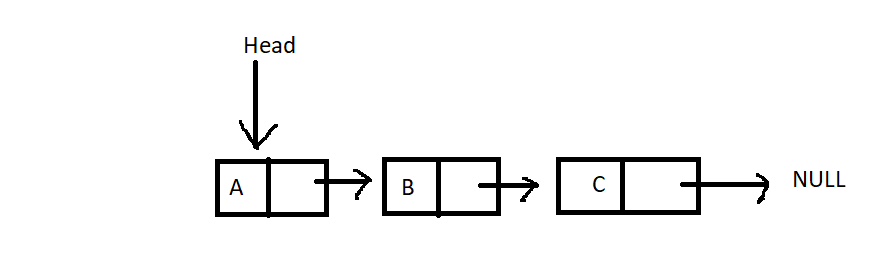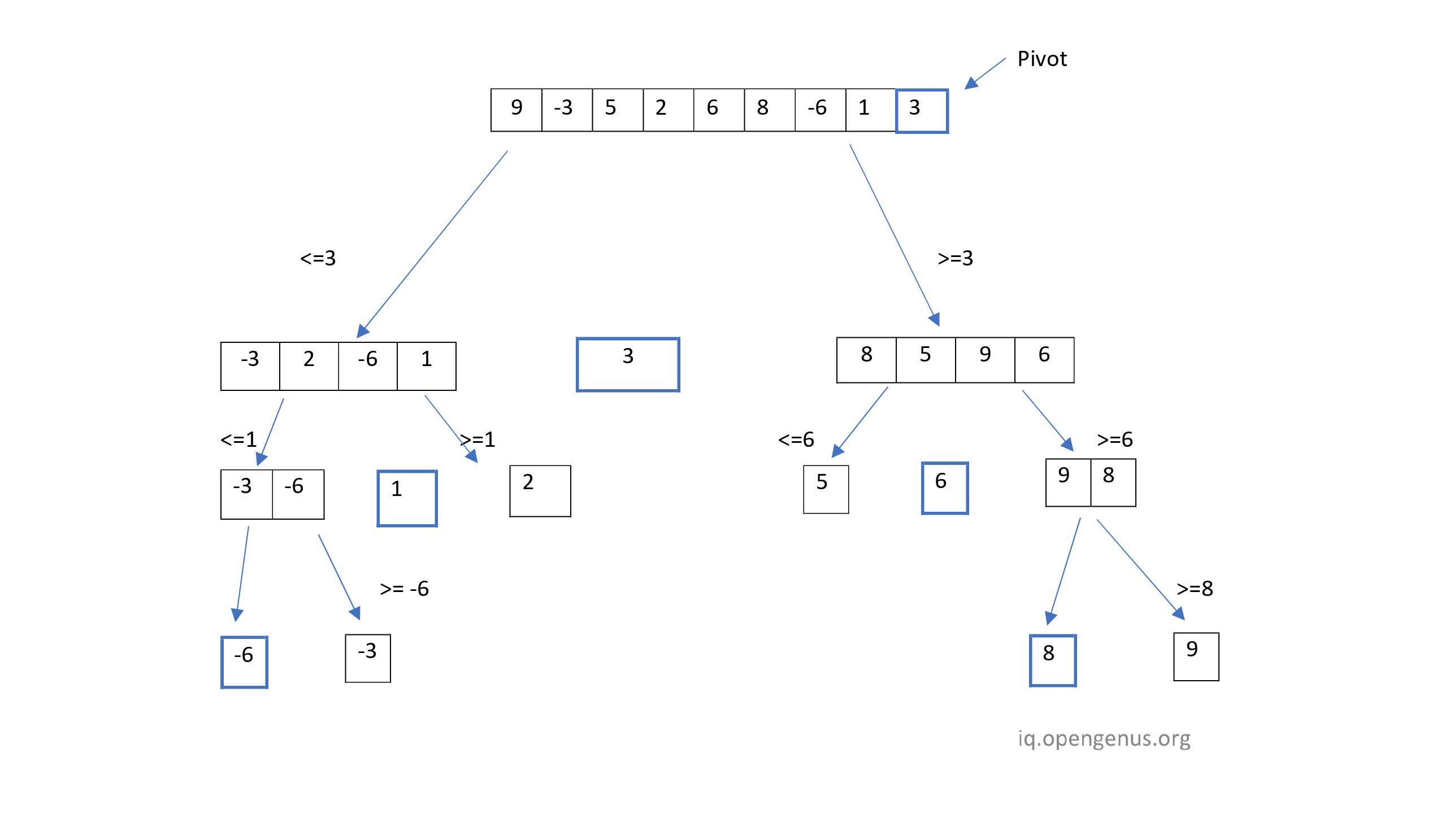×

Search anything:

# Quick Sort on Linked List

#### Algorithms Sorting Algorithms linked listGet this book -> Problems on Array: For Interviews and Competitive Programming

In this article, we have explained how to implement Quick Sort on Linked List and if it is as efficient as Quick Sort on Array.

2. Introduction to Quick Sort
4. QuickSort on Linked List vs Array
5. Time and Space Complexity of Quick Sort on Linked List
6. Conclusion

Before we begin discussing how to perform quicksort on linked lists, let's define what a linked list is and what quicksort is.

Linked lists are linear data structures that are used to store data. However, unlike arrays, linked lists don't store their elements in consecutive memory locations.
The database is an efficient way to store data when you don't know how many elements will be in the list at any given time. Constant insertion and deletion are possible.
Linked lists typically contain two fields:

• Data Field - To store data.
• Next Pointer - To store the next element's location.A Head Pointer is used to point to the first node of a linked list, with the head pointer we can access all the elements of the list.

Various types of Linked Lists are:

2. Doubly Linked List. ( Has Prev pointer to access previous element)
3. Circular Linked List. (Circular in Nature , can traverse the whole list by starting at any point)

# Introduction to Quick Sort

Sorting is the process of ordering elements in ascending or descending order based on some relationship among the data items.This is an efficient sorting algorithm that sorts arrays. It is a Divide and Conquer algorithm which, as the name suggests, divides the problem into smaller subproblems until each subproblem is simple enough to be solved easily.QuickSort works by picking a pivot element from the array, placing that element at its correct place, and then placing smaller elements before it and larger elements after it , the partition() method is used to perform this process.The Pivot can be Picked as the first , last element or can be a random element.
Time Complexity of QuickSort is O(nlogn)

QuickSort in LinkedLists works by using the last element of the list as the pivot Element. The Logic is Divided into 2 methods , they are:

• QuickSort : This method is used to recursively call itself until the List is sorted. It has a call for the method PartitionLast , which is used to partition the list based on the last element of the list as the pivot element.After this method call , the list is subdivided into 2 parts , the part before the pivot element and the part after the pivot element , these are sorted based on the last element of these lists as pivot element. First the Left part is sorted , then the right.
• PartitionLast : This method is used to place the pivot element , which is the last element of the list at it's sorted position. This works by placing all the elements which are smaller than the pivot to it's left and all the elements larger than the pivot at the tail of the list. Returns the element before the pivot.

QuickSort Implementation in Java.

``````class Node{
int data;
Node next;
Node(int data){
this.data= data;
}
}

class Quicksort{

QuickSort(){
}

{
else
{
while(temp.next != null)
temp = temp.next;
temp.next = new Node(data);
}
}

void printList(){
System.out.println();
while(temp != null)
{
System.out.print(temp.data + " -> ");
}
System.out.print("NULL");
}

Node paritionLast(Node start, Node end)
{
if (start == end || start == null || end == null)
return start;

Node pivot_prev = start;
Node curr = start;
int pivot = end.data;

// iterate till one before the end,
// no need to iterate till the end
// because end is pivot
while (start != end) {
if (start.data < pivot) {
pivot_prev = curr;
int temp = curr.data;
curr.data = start.data;
start.data = temp;
curr = curr.next;
}
start = start.next;
}

// swap the position of curr i.e.
// next suitable index and pivot
int temp = curr.data;
curr.data = pivot;
end.data = temp;

// return one previous to current
// because current is now pointing to pivot
return pivot_prev;
}

void sort(Node start, Node end)
{
if(start == null || start == end|| start == end.next )
return;

// split list and partition recurse
Node pivot_prev = paritionLast(start, end);
sort(start, pivot_prev);

// if pivot is picked and moved to the start,
// that means start and pivot is same
// so pick from next of pivot
if (pivot_prev != null && pivot_prev == start)
sort(pivot_prev.next, end);

// if pivot is in between of the list,
// start from next of pivot,
// since we have pivot_prev, so we move two nodes
else if (pivot_prev != null
&& pivot_prev.next != null)
sort(pivot_prev.next.next, end);
}

public static void main(String[] args)
{
QuickSort quick = new QuickSort();
System.out.println("Before Sorting");
quick.printList();
System.out.println("After Sorting");
quick.sort();
quick.printList();

}

}
``````

Output

``````Before Sorting

5 -> 2 -> 0 -> 10 -> 9 -> NULL
After Sorting

0 -> 2 -> 5 -> 9 -> 10 -> NULL
``````

As you can see , we have a sorted list after running QuickSort on the List.

# QuickSort on Linked List vs Array

QuickSort can be implemented both on Arrays as well as on Linkedlists.

• In Arrays,all the elements are stored in contiguous memory locations, O(1) constant access to all the elements is possible , as quicksort is an In-place algorithm , it is the preferred sorting algorithm as there is no auxillary space required for sorting the elements. The worst case scenario for quicksort is O(N^2) , which can be avoided by picking a random pivot.

• In LinkedLists , the average time to access each element is O(n) , which is not constant . The benefits of this being In-place Vanishes in LinkedLists.
QuickSort in LinkedLists works by swapping the content of the nodes rather than the nodes. The Partition Method divides the list into 2 parts based on the pivot element. These 2 parts usually aren't of the same size as we cannot guarantee that the pivot element will divide the list into 2 equal parts. Picking the last element of the list is usually done because picking a random pivot from the lists would incur a penalty as access is not constant. As the pivot selected is the usually the last element , depending on the pattern of data , we could easily hit the worst case scenario.

# Time and Space Complexity of Quick Sort on Linked List

• On Arrays
Time Complexity
1. Best Case : O(NlogN)
2. Average Case : O(NLogN)
3. Worst Case : O(N^2)

Space Complexity : O(1)

1. Best Case : O(NLogN)
2. Average Case : O(NlogN)
3. Worst Case : O(N^2)

Space Complexity : O(1)

# Conclusion

QuickSort is an Optimal Sorting algorithm which can be used on arrays as well as on linked lists with little modification. In Arrays,it is an In-place sorting algorithm , it uses constant space and its Time complexity is O(NlogN) , where N is the number of nodes in the list.

On LinkedLists , QuickSort can be very efficient but the probability of hitting the worst case scenario will also increase because it uses a predefined pivot.Randomization can help mitigate this issue but will incur a penalty which increases runtime , that's why MergeSort is preferred in LinkedLists because it will guarantee a worst case runtime of O(NlogN) and also the space complexity of MergeSort is constant for LinkedLists.

With this article at OpenGenus, you must have the complete idea of Quick Sort on Linked List.#### Mohd Ehtesham Uddin Qureshi

Mohd Ehtesham Uddin Qureshi completed B.E in Computer Science from Muffakham Jah College of Engineering and Technology (2021). He is an Intern at OpenGenus.